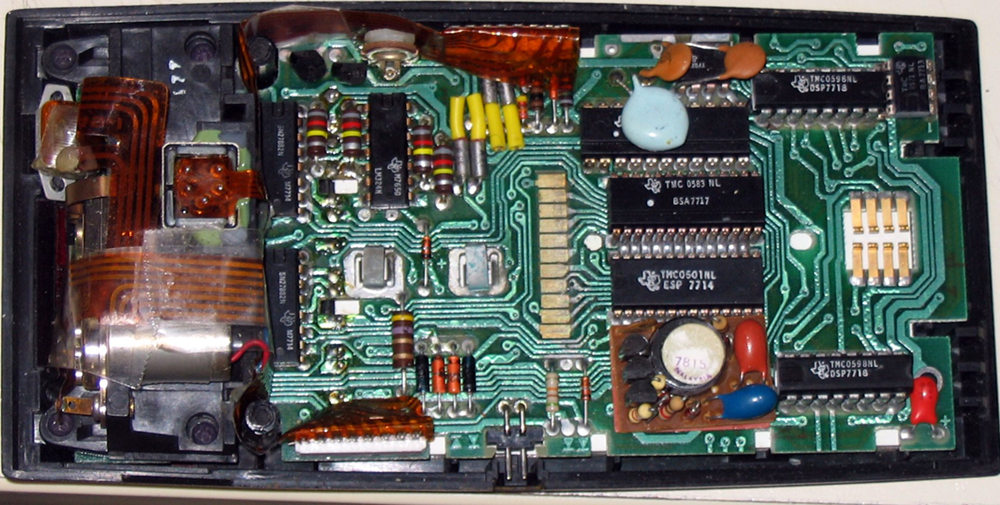# 19.7 Energy stored in capacitors

 Page 1 / 3
• List some uses of capacitors.
• Express in equation form the energy stored in a capacitor.
• Explain the function of a defibrillator.

Most of us have seen dramatizations in which medical personnel use a defibrillator    to pass an electric current through a patient’s heart to get it to beat normally. (Review [link] .) Often realistic in detail, the person applying the shock directs another person to “make it 400 joules this time.” The energy delivered by the defibrillator is stored in a capacitor and can be adjusted to fit the situation. SI units of joules are often employed. Less dramatic is the use of capacitors in microelectronics, such as certain handheld calculators, to supply energy when batteries are charged. (See [link] .) Capacitors are also used to supply energy for flash lamps on cameras.Energy stored in the large capacitor is used to preserve the memory of an electronic calculator when its batteries are charged. (credit: Kucharek, Wikimedia Commons)

Energy stored in a capacitor is electrical potential energy, and it is thus related to the charge $Q$ and voltage $V$ on the capacitor. We must be careful when applying the equation for electrical potential energy $\text{Δ}\text{PE}=q\text{Δ}V\phantom{\rule{0.25em}{0ex}}$ to a capacitor. Remember that $\text{Δ}\text{PE}$ is the potential energy of a charge $q$ going through a voltage $\text{Δ}V$ . But the capacitor starts with zero voltage and gradually comes up to its full voltage as it is charged. The first charge placed on a capacitor experiences a change in voltage $\text{Δ}V=0$ , since the capacitor has zero voltage when uncharged. The final charge placed on a capacitor experiences $\text{Δ}V=V$ , since the capacitor now has its full voltage $V$ on it. The average voltage on the capacitor during the charging process is $V/2$ , and so the average voltage experienced by the full charge $q$ is $V/2$ . Thus the energy stored in a capacitor, ${E}_{\text{cap}}$ , is

${E}_{\text{cap}}=\frac{QV}{2},$

where $Q$ is the charge on a capacitor with a voltage $V$ applied. (Note that the energy is not $\text{QV}$ , but $\text{QV}/2$ .) Charge and voltage are related to the capacitance $C$ of a capacitor by $Q=\text{CV}$ , and so the expression for ${E}_{\text{cap}}$ can be algebraically manipulated into three equivalent expressions:

${E}_{\text{cap}}=\frac{\text{QV}}{2}=\frac{{\text{CV}}^{2}}{2}=\frac{{Q}^{2}}{2C},$

where $Q$ is the charge and $V$ the voltage on a capacitor $C$ . The energy is in joules for a charge in coulombs, voltage in volts, and capacitance in farads.

## Energy stored in capacitors

The energy stored in a capacitor can be expressed in three ways:

${E}_{\text{cap}}=\frac{\text{QV}}{2}=\frac{{\text{CV}}^{2}}{2}=\frac{{Q}^{2}}{2C},$

where $Q$ is the charge, $V$ is the voltage, and $C$ is the capacitance of the capacitor. The energy is in joules for a charge in coulombs, voltage in volts, and capacitance in farads.

In a defibrillator, the delivery of a large charge in a short burst to a set of paddles across a person’s chest can be a lifesaver. The person’s heart attack might have arisen from the onset of fast, irregular beating of the heart—cardiac or ventricular fibrillation. The application of a large shock of electrical energy can terminate the arrhythmia and allow the body’s pacemaker to resume normal patterns. Today it is common for ambulances to carry a defibrillator, which also uses an electrocardiogram to analyze the patient’s heartbeat pattern. Automated external defibrillators (AED) are found in many public places ( [link] ). These are designed to be used by lay persons. The device automatically diagnoses the patient’s heart condition and then applies the shock with appropriate energy and waveform. CPR is recommended in many cases before use of an AED.

What is specific heat capacity?
Specific heat capacity is the amount of heat required to raise the temperature of one (Kg) of a substance through one Kelvin
Paluutar
formula for measuring Joules
I don't understand, do you mean the S.I unit of work and energy?
hamidat
what are the effects of electric current
What limits the Magnification of an optical instrument?
Lithography is 2 micron
Venkateshwarlu
what is expression for energy possessed by water ripple
what is hydrolic press
An hydraulic press is a type of machine that is operated by different pressure of water on pistons.
hamidat
what is dimensional unite of mah
i want jamb related question on this asap🙏
What is Boyles law
it can simple defined as constant temperature
Boyles law states that the volume of a fixed amount of a gas is inversely proportional to the pressure acting on in provided that the temperature is constant.that is V=k(1/p) or V=k/p
what is motion
getting notifications for a dictionary word, smh
Anderson
what is escape velocity
the minimum thrust that an object must have in oder yo escape the gravitational pull
Joshua
what is a dimer
Mua
what is a atom
how to calculate tension
what are the laws of motion
Mua
what is force
Force is any quantity or a change that produces motion on an object body.
albert
A force is a push or a pull that has the tendency of changing a body's uniform state of rest or uniform state of motion in a straight line.
nicholas
plsoo give me the gravitational motion formulas
Okoye
f=Gm/d²
What is the meaning of emf
electro magnetic force
shafiu
Electromotive force (emf) is a measurement of the energy that causes current to flow through a circuit.
tritium (gas, netrogen, cloud, lamp)
Continue - > tritium (gas, netrogen, cloud, lamp span, lamp light, cool)
firdausByBy RhodesByByByBy MldelatteByBy